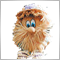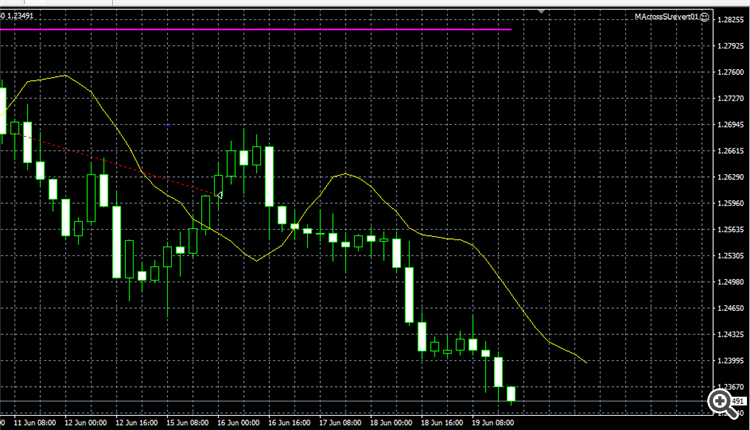62

Hi,

Need help explaining why my EA skips trades when running on live account. When backtesting the same period, it is clear that certain trades are not taken when trading live. Does anyone know why this might happen? To be clear, the parameters are the same.

Does anyone have any tips or pointers as to what the problem is? How to debug/solve it?

Appreciate all help!Moderator
234459

Hi,

Need help explaining why my EA skips trades when running on live account. When backtesting the same period, it is clear that certain trades are not taken when trading live. Does anyone know why this might happen? To be clear, the parameters are the same.

Does anyone have any tips or pointers as to what the problem is? How to debug/solve it?

Appreciate all help!

The main reason is errors in your code.

If you want to be helped in your MQL5 code, attach the source (editable) MQL5 code. Attach screenshots of the chart and indicate on which site (when working online) there was no transaction.62

Thanks for a very quick response. The expert is in mql4 and not mql5. I hope you can still help me. I am attaching the EA with this message. What do you mean y which site? If you mean the broker, then it is tradeview. Thanks very much in advance.Below is the backtesting

Files:62

```//+------------------------------------------------------------------+
//|                                    MACrossSLrevert01  EAV1.mq4   |
//|      Change: Similar to MAcrossover with some differences:       |
//|             1- Only one MA is used that follows CLOSE            |
//|             2- A slow MA is used to identify the trend           |
//|             3- Only Buy/Sell when above/below slow MA            |
//|             4- Buy/Sell when reversing from touching Dn/Up SL    |
//+------------------------------------------------------------------+
#property version   "1.01"
#property strict

input bool   OpenSELL=True;
input bool   CloseBySignal=True;
input int SLtype=2;
// 0 for constant pips or none, 1 for most recent high/low, 2 for multiples of ATR, 3 combi
input double StopLoss=4.0;
// Depending on type: 0 none, or used as multiple of what type was chosen
input int SLlkbk=0;
// Look back in bars for stop loss. Used only with types 1 and 3
input double MaxSLPips = 0;
// The maximum allowed SL. If zero then none. Else SL may not exceed the max.
input int ProfitType = 0;
// 0 for pips, 1 for ATR,
input double TakeProfit=0;
// if 0 no profit taking, pips if ProfitType 0, multiples of ATR if ProfitType 1
input double TrailingStop=5.0;
// if 0 no trailing stop, otherwise multiples of ATR
input int SlowPeriod = 1200;
// for H4 which translates to 200 days. 12 translates to 2 days and shift=6 to the average of yesterday
input int Fastperiod = 12;
input int Lag = 6;
input int ATRPeriod=20;
input int SLPeriod = 20;
input double delta = -0.3;
input bool   AutoLot=False;
input int RiskMethod = 3;
//1 for constant risk percentage, 2 variable risk
input double MinRisk=0.1;
input double MaxRisk=2;
input double ManualLots=0.1;
input int    MagicNumber=73456234;
input string Koment="EA-TrendFollow03";
input int    Slippage=10;
input double modifstep = 5;

//---
int ticket;
int LotDigits;
double Trail,iTrailingStop;
double Risk;
int tmfrm;
double SLpoints = 0;
datetime Now1 = 0;
datetime Now2 = 0;
datetime tbarTime = 0;
//+------------------------------------------------------------------+
//| Expert initialization function                                   |
//+------------------------------------------------------------------+
int init()
{
tmfrm = Period();
Now1 = Time;
Now2 = Time;
return(0);
}
//+------------------------------------------------------------------+
//|                                                                  |
//+------------------------------------------------------------------+
int deinit()
{
return(0);
}
//+------------------------------------------------------------------+
//|                                                                  |
//+------------------------------------------------------------------+
int start()
{
bool SigDn=False, SigUp=False;
double stoplevel=MarketInfo(Symbol(),MODE_STOPLEVEL);
double minstoplevel = MarketInfo( Symbol(), MODE_STOPLEVEL );
double atr = 0, slowAvg = 0, MA1 = 0, MA2 = 0;
//   double oldHigh, oldLow, newHigh, newLow;
double newHigh, newLow;
bool static lower, higher;
//   double std, dd;

//   if(Time != Now1)
//   {
Now1 = Time;
slowAvg = iMA(NULL,0,SlowPeriod,0,MODE_SMA,PRICE_CLOSE,1);
MA1 = iMA(NULL, 0, Fastperiod, Lag, MODE_SMA, PRICE_CLOSE, 1);
MA2 = iMA(NULL, 0, Fastperiod, Lag, MODE_SMA, PRICE_CLOSE, 2);
atr = iATR(NULL,0,ATRPeriod,1);
//      oldLow = iCustom(NULL, 0, "StopLossDn", delta, 0, SLPeriod, ATRPeriod,0,2);
newLow = iCustom(NULL, 0, "StopLossDn", delta, 0, SLPeriod, ATRPeriod,0,1);
//      oldHigh = iCustom(NULL, 0, "StopLossUp", delta, 0, SLPeriod, ATRPeriod,0,2);
newHigh = iCustom(NULL, 0, "StopLossUp", delta, 0, SLPeriod, ATRPeriod,0,1);
//   }

if( Now2 != Time )//Is it a new bar candle?
{
Now2 = Time;
//    Calculate the risk (beginning of bar, each bar)
if(RiskMethod == 2)
{
Risk = CoeffSize();
}
else Risk = MaxRisk;
}

OrderSell=0;
for(int cnt=0; cnt<OrdersTotal(); cnt++)
{
{
if(OrderSymbol()==Symbol() && OrderMagicNumber()==MagicNumber && OrderComment()==Koment)
{
if(OrderType()==OP_SELL) OrderSell++;
{
}
if(OrderType()==OP_SELL)
{
if(OrderStopLoss() > ts1sell + modifstep*Point)
ticket=OrderModify(OrderTicket(),OrderOpenPrice(),ts1sell,OrderTakeProfit(),0,Blue);
}
}
}
}
if(Close > MA1 && Close < MA2)
{
SigUp = True;
SigDn = False;
//      Print("SigUp H1", High, " L1 ", Low, " Up ", Up, " Dn ", Dn, " Ask ", Ask, " DnPast ", DnPast, " UpPast ", UpPast, " H2 ", High, " L2 ", Low);
}
if(Close < MA1 && Close > MA2)
{
SigDn = True;
SigUp = False;
//      Print("SigDn H1", High, " L1 ", Low, " Up ", Up, " Dn ", Dn, " Bid ", Bid, " DnPast ", DnPast, " UpPast ", UpPast, " H2 ", High, " L2 ", Low);
}
if(Low < newLow)
{
lower = True;
higher = False;
}
else if(High > newHigh)
{
higher = True;
lower = False;
}

//   if(OpenSELL && OrderSell<MaxNoTrades && SigDn && Close < slowAvg && higher && tbarTime != Time)
if(OpenSELL && OrderSell<MaxNoTrades && SigDn && higher && tbarTime != Time)
{
tbarTime = Time;
//      Print("SigDn High ", High, " Low ", Low, " Up ", Up, " Dn ", Dn, " Bid ", Bid, " Mid ", Mid, " MidP ", MidPast, " ma ", movAvg);
OPSELL();
}
{
tbarTime = Time;
//      Print("SigDn High ", High, " Low ", Low, " Up ", Up, " Dn ", Dn, " Bid ", Ask, " Mid ", Mid, " MidP ", MidPast, " ma ", movAvg);
}
//--- close position by signal
if(CloseBySignal)
{
if(OrderSell>0 && SigUp) CloseSell();
}
//---
return(0);
}
//+------------------------------------------------------------------+
//|                                                                  |
//+------------------------------------------------------------------+
{
double StopLossLevel;
double TakeProfitLevel;
switch(SLtype)
{
case 0:  if(StopLoss>0)
{
StopLossLevel=Bid-StopLoss*Point;
}
else
{
StopLossLevel=0.0;
}
break;
case 1:  StopLossLevel=iLow(NULL, 0, iLowest(NULL, 0, MODE_LOW, SLlkbk, 0))-0.1*iATR(NULL,0,ATRPeriod,1); SLpoints = Bid-StopLossLevel; break;
case 2:  StopLossLevel=Bid-iATR(NULL,0,ATRPeriod,1)*StopLoss; SLpoints = Bid-StopLossLevel; break;
case 3:  StopLossLevel=MathMin(iLow(NULL, 0, iLowest(NULL, 0, MODE_LOW, SLlkbk, 0))-0.1*iATR(NULL,0,ATRPeriod,1),Bid-iATR(NULL,0,ATRPeriod,1)*StopLoss); SLpoints = Bid-StopLossLevel; break;
default: Print("Error opening BUY order : ",GetLastError()," ",SLtype);
}
//   Print(Close," ",StopLossLevel, " ", SLtype);
if(TakeProfit>0)
switch(ProfitType)
{
default: Print("Error opening BUY order : ",GetLastError()," ",ProfitType);
}
else TakeProfitLevel=0.0;

if (MaxSLPips > 0) {
if (Bid - StopLossLevel > MaxSLPips*Point) StopLossLevel = Bid - MaxSLPips*Point;
//      if (Bid - StopLossLevel > MaxSLPips*Point) return;
//      if (Bid - iLow(NULL, 0, iLowest(NULL, 0, MODE_LOW, SLlkbk, 0)) > MaxSLPips*Point) return;
//      Print("MaxSLPips ", MaxSLPips, " StopLossLevel ", StopLossLevel, " Bid ", Bid, " low ", iLow(NULL, 0, iLowest(NULL, 0, MODE_LOW, SLlkbk, 0)));
}
//   StopLossLevel = MathMax(StopLossLevel,MarketInfo(Symbol(),MODE_STOPLEVEL));
//   Print("Buy ", StopLossLevel, " ", Bid);
//   {
//   }
//   Print("Buy ", "SigUp ", SigUp, " SigDn ", SigDn, " a1 ", a1, " a2 ", a2);
}
//+------------------------------------------------------------------+
//|                                                                  |
//+------------------------------------------------------------------+
void OPSELL()
{
double StopLossLevel;
double TakeProfitLevel;
switch(SLtype)
{
case 0:  if(StopLoss>0)
{
}
else
{
StopLossLevel=0.0;
}
break;
case 1:  StopLossLevel=iHigh(NULL, 0, iHighest(NULL, 0, MODE_HIGH, SLlkbk, 0))+0.1*iATR(NULL,0,ATRPeriod,1); SLpoints = StopLossLevel-Ask; break;
case 3:  StopLossLevel=MathMax(iHigh(NULL, 0, iHighest(NULL, 0, MODE_HIGH, SLlkbk, 0))+0.1*iATR(NULL,0,ATRPeriod,1),Ask+iATR(NULL,0,ATRPeriod,1)*StopLoss); SLpoints = StopLossLevel-Ask; break;
default: Print("Error opening BUY order : ",GetLastError()," ",SLtype);
}
//   Print(Close," ",StopLossLevel, " ", SLtype);
if(TakeProfit>0)
switch(ProfitType)
{
case 0: TakeProfitLevel=Bid-TakeProfit*Point;break;
case 1: TakeProfitLevel=Bid-TakeProfit*iATR(NULL,0,ATRPeriod,1);break;
//         case 2: TakeProfitLevel=Bid-TakeProfit*Bdev*iATR(NULL,0,ATRPeriod,1);break;
default: Print("Error opening SELL order : ",GetLastError()," ",ProfitType);
}
else TakeProfitLevel=0.0;
//---

if (MaxSLPips > 0) {
//      if (StopLossLevel - Ask > MaxSLPips*Point) return;
//      if (iHigh(NULL, 0, iHighest(NULL, 0, MODE_HIGH, SLlkbk, 0)) - Ask > MaxSLPips*Point) return;
//      Print("MaxSLPips ", MaxSLPips, " StopLossLevel ", StopLossLevel, " Ask ", Ask);
}
//   StopLossLevel = MathMin(StopLossLevel,MarketInfo(Symbol(),MODE_STOPLEVEL));
//   Print("Sell ", StopLossLevel, " ", Ask);
ticket=OrderSend(Symbol(),OP_SELL,LOT(),Bid,Slippage,StopLossLevel,TakeProfitLevel,Koment,MagicNumber,0,DeepPink);
//   {
//      ticket=OrderSend(Symbol(),OP_SELL,LOT(),Bid,Slippage,StopLossLevel,TakeProfitLevel,Koment,MagicNumber,0,DeepPink);
//   }
//   Print("Sell ", "SigUp ", SigUp, " SigDn ", SigDn, " a1 ", a1, " a2 ", a2);
}
//+------------------------------------------------------------------+
//|                                                                  |
//+------------------------------------------------------------------+
void CloseSell()
{
int  total=OrdersTotal();
for(int y=OrdersTotal()-1; y>=0; y--)
{
if(OrderSymbol()==Symbol() && OrderType()==OP_SELL && OrderMagicNumber()==MagicNumber)
ticket=OrderClose(OrderTicket(),OrderLots(),OrderClosePrice(),5,Black);
//           {
//            ticket=OrderClose(OrderTicket(),OrderLots(),OrderClosePrice(),5,Black);
//           }
}
}
//+------------------------------------------------------------------+
//|                                                                  |
//+------------------------------------------------------------------+
{
int  total=OrdersTotal();
for(int y=OrdersTotal()-1; y>=0; y--)
{
ticket=OrderClose(OrderTicket(),OrderLots(),OrderClosePrice(),5,Black);
//           {
//            ticket=OrderClose(OrderTicket(),OrderLots(),OrderClosePrice(),5,Black);
//           }
}
}

//+------------------------------------------------------------------+
//| Calculates position size                                         |
//+------------------------------------------------------------------+
double CoeffSize()
{
double Loss=1,Profit=1,Prcnt,risk;

Profit = 0;
Loss = 0;
Risk = MinRisk;
else
{
for (Index = OrdersHistoryTotal() - 1; Index >= 0; Index--) {
if(OrderSelect(Index, SELECT_BY_POS, MODE_HISTORY))
{
if((OrderSymbol() == Symbol()) && (OrderMagicNumber() == MagicNumber) && (trades <= NumTrades)) {
if(OrderProfit() <= 0) {
Loss = Loss - OrderProfit()/OrderLots();  // Add up our previous losses
//               Size = 1 + MathRound(0.5 + Loss / (ProfitPerPip * Lots * ProfitTarget));
}
else if(OrderProfit() > 0)
{
Profit = Profit + OrderProfit()/OrderLots();  // Add up our previous losses
}
//            else {
//               break;                        // Found a profitable trade, so we're all done
//            }

}
}
}
}
if(Profit != 0 || Loss != 0)
{
Prcnt = Profit/(Profit+Loss);
risk = Prcnt*MaxRisk+(1-Prcnt)*MinRisk;
}
else risk = 0.5*(MaxRisk+MinRisk);
Print("Loss = ", Loss, ", Profit = ", Profit, ", Risk = ", risk);
return (risk);
}

//+------------------------------------------------------------------+
//|                                                                  |
//+------------------------------------------------------------------+
double LOT()
{
double lotsi;
double ilot_max =MathMin(MarketInfo(Symbol(),MODE_MAXLOT),ManualLots);
double ilot_min =MarketInfo(Symbol(),MODE_MINLOT);
double tick=MarketInfo(Symbol(),MODE_TICKVALUE);
//---
double  myAccount=AccountBalance();
//---
if(ilot_min==0.01) LotDigits=2;
if(ilot_min==0.1) LotDigits=1;
if(ilot_min==1) LotDigits=0;
//---
if(AutoLot && RiskMethod != 3)
{
lotsi=NormalizeDouble((myAccount*Risk)/10000,LotDigits);
}
else if(AutoLot && RiskMethod ==3)
{
int SLpips = MathRound(SLpoints*10000);
lotsi = CalcLotSize(SLpips,Risk);
Print(SLpoints, " ", SLpips, " ", lotsi);
}
else
{
lotsi=ManualLots;
}
//---
if(lotsi>=ilot_max) { lotsi=ilot_max; }
//---
return(lotsi);
}

//-----------------------------------------------------------------------------
// function: CalcLotSize()
// Description: Calc Lot Size based on risk setting
//-----------------------------------------------------------------------------
double CalcLotSize(int iSL,double dRisk)
{
double dBalance;
double dRiskCapital;

dBalance=AccountFreeMargin();
dRiskCapital=dRisk/100*dBalance;
//  return( dRiskCapital/(iSL*MarketInfo(Symbol(),MODE_TICKVALUE)*iPoint));
Print(iSL, " ", MODE_TICKVALUE, " ", MarketInfo(Symbol(), MODE_TICKVALUE));
return( dRiskCapital/(iSL*MarketInfo(Symbol(),MODE_TICKVALUE)*10));
}
```190

During backtesting did you set the modelling to be "every tick based on real ticks"?62

WindmillMQL:
During backtesting did you set the modelling to be "every tick based on real ticks"?

@WindmillMQL, Yes!24283

omar_askary: The expert is in mql4 and not mql5.

Why did you post your MT4 question in the MT5 EA section instead of the MQL4 section, (bottom of the Root page?)
General rules and best pratices of the Forum. - General - MQL5 programming forum?
Next time post in the correct place. The moderators will likely move this thread there soon.62

William Roeder:

Why did you post your MT4 question in the MT5 EA section instead of the MQL4 section, (bottom of the Root page?)
General rules and best pratices of the Forum. - General - MQL5 programming forum?
Next time post in the correct place. The moderators will likely move this thread there soon.

@William Roeder

Thank you for pointing that out for me. The title of the section is "automated trading ..." without any restrictions to what mql4, therefrom is my mistake.